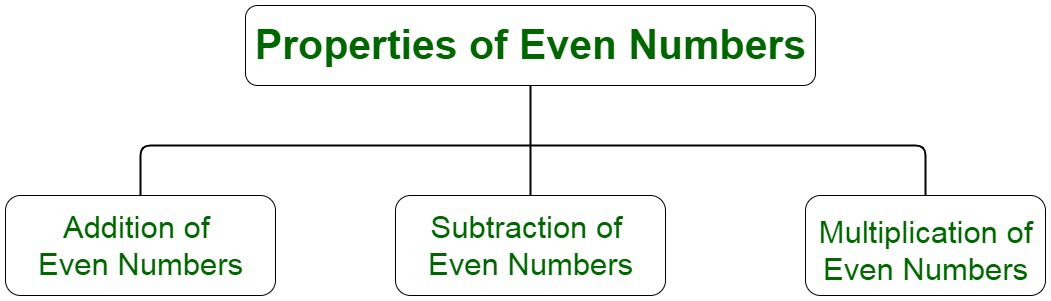Open in App
Not now

# Find the mean of first 10 even numbers

• Last Updated : 06 Oct, 2021

An even number is a number that is divisible by 2. Upon division of this number into equivalent groups, no number is left as a remainder. An even number is considered to be a multiple of 2. All the even numbers, therefore, can be paired into groups leaving no items unpaired. When divided by 2, the remainder returned is 0.

### Properties of Even NumbersSome of the basic properties of even numbers are as follows:

• The sum of two even numbers is an even number. For instance, the sum of numbers of 2 and 4 is 6.
• The sum of an even number and an odd number is an odd number. For instance, the sum of numbers of 2 and 5 is 7.
• The sum of two odd numbers is an even number. For instance, the sum of numbers of 3 and 5 is 8.

Subtraction of Even Numbers:

• The difference between two even numbers is also an even number. The result, however, may be positive or negative. For instance, the difference between two numbers 4 and 2 is 2.
• The difference between an even number and an odd number is an odd number. For instance, the difference of 15 and 4 = 15-4 = 11.

Multiplication of Even Numbers:

• The multiplication of two even numbers is an even number. For instance, the multiplication of numbers of 2 and 4 is 8.
• The multiplication of two odd numbers is an odd number. For instance, the multiplication of numbers of 3 and 5 is 15.
• The multiplication of an even and an odd number is an even number. For instance, the multiplication of numbers of 2 and 3 is 6.

### Mean

Mean is one of the statistical concepts which is used to calculate the average or a central value of a given set of integers. It gives information about the central tendency of the data. The central tendency of the data is a statistical measure that uses a single value to gather information about the entire set of data distribution. It provides a description of the entire data set. Mean is equivalent to the sum of all observations to the total number of observations. Mean is also called the arithmetic mean or average. Let us assume a data set to be given by, X = x1, x2,…, xn. The mean is denoted by x̄  which is the mean of given n values.

According to the definition, we have,

X̄ = (Sum of values ÷ Number of values)

X̄ = (x1​+x2​+x3​+….+xn​)​ / n

Some of the examples of mean in our real life are:

• Mean of marks scored by students in a test.
• Mean of runs scored by a cricketer in a one day match.

### Computing Mean

The mean of the data set can be calculated by computing the average of the numbers. In the case of negative numbers, the summation of data values is calculated and then divided by the number of negative integers.

For instance, if we have four random integer values, such as -2,0,4,5 , we can compute mean by calculating the average of these numbers, which is given by,

Average = Sum / Number of such values

Sum = -2 + 0 + 4 + 5

= 7

And, the number of values = 4

Average = 7/4 = 3

Therefore, the mean of these values is equivalent to 7/4.

### What is the mean of the first 10 even numbers?

Natural numbers are counting numbers beginning from 1.

The first 10 even natural numbers, therefore begin with 2.

Hence, we have the first 10 natural numbers to be 2, 4, 6, 8, 10, 12, 14, 16, 18, 20.

Sum of numbers = 2 + 4… + 20 = 110

Now, we have,

Mean = Sum of numbers / Count of numbers

Substituting the values, we get,

Mean = 110 / 10 = 11

Therefore, the required mean of the first 10 even natural numbers is equivalent to 11.

### Similar Questions

Question 1: How does the computation of the first 10 even natural numbers form an AP?

Sum (S) = (a+l) n/2

where a is the first term in the series, l is the last term of the series and n is the number of terms.

Substituting the values, we get,

a = 2, l = 20, n = 10 we get,

S = (2+20) 10/2

We obtain,

S = 5 × (22)

= 110

Question 2: The mean of 4 even numbers is 6. Two of them are 2 and 4. The remaining two are 3x and x+2. Calculate the third and fourth numbers.

Sum of numbers = 2 + 4 + 3x + x+2 = 2x + 8

Mean = (4x + 8)/4

6 = 2x + 4

2 = 2x

x = 1

Question 3: Compute the mean of the first 50 even numbers.

Since, we have, first 50 even natural numbers are 2,4,6,………,98,100.

Now, we have,

Mean = Sum of observation / Number of observations

Sum of observation = 2+4+6……+98+100 = 2(1+2+3……+50)

We know,

Sum of n natural number = n(n+1) /2

On substituting the values, we get,

So, (1+2+3……+50) = 50 × 51/2

Sum = 2 × 50 × (51 / 2)

= 50 × 51

Total no. of such observation = 50

Therefore,

Mean = 50 × (51 / 50)

= 51

My Personal Notes arrow_drop_up